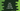# 5 Different ways in Dart to iterate through a Map# Dart programs to iterate through a map:

A `Map` in Dart is used to hold key-value pairs. We can have any type of key or value. The key should be unique. We can’t have two equal keys for a dart map. But we can have multiple values with the same content.

You can iterate through the key-value pairs, or only through the keys or only through the values. We can use forEach and for-in loop for the iteration. In this post, I am listing down a couple of different ways to do that.

### 1. forEach to iterate key-value pairs of a dart map:

The forEach function takes one function as its parameter in dart. You can use two parameters in the function to get both key and value of a map:

``````main() {
var numMap = Map();
numMap["one"] = 1;
numMap["two"] = 2;
numMap["three"] = 3;
numMap["four"] = 4;
numMap["five"] = 5;

numMap.forEach((k, v) => print("Key : \$k, Value : \$v"));
}``````

Here, k and v are the key and value of the element it is iterating. It will print all keys and values of numMap:

``````Key : one, Value : 1
Key : two, Value : 2
Key : three, Value : 3
Key : four, Value : 4
Key : five, Value : 5``````

### 2. for-in to iterate keys and values :

You can get the value of a dart map pair if you know the key associated with that value. We can use `map[key]` to get the value for the `key` in the `map`. The `keys` property of a Dart map returns all the keys of the map.

By using one for-in loop, we can iterate through the keys and for each key, we can get the value:

``````main() {
var numMap = Map();
numMap["one"] = 1;
numMap["two"] = 2;
numMap["three"] = 3;
numMap["four"] = 4;
numMap["five"] = 5;

for (var k in numMap.keys) {
print("Key : \$k, value : \${numMap[k]}");
}
}``````

The output is similar to the above example.

### 3. forEach to print key-values using keys :

By using the forEach loop, we can iterate only through the keys and for each key, we can print the value:

``````main() {
var numMap = Map();
numMap["one"] = 1;
numMap["two"] = 2;
numMap["three"] = 3;
numMap["four"] = 4;
numMap["five"] = 5;

numMap.keys.forEach((k) => print("Key : \$k, Value : \${numMap[k]}"));
}``````

We are iterating over the keys returned by the `keys` property of the map `numMap`.

### 4. Iterate through the values:

The values property of Dart map returns one iterable holding the map values. You can use one forEach or for-in to iterate through these values. Example using for in:

``````main() {
var numMap = Map();
numMap["one"] = 1;
numMap["two"] = 2;
numMap["three"] = 3;
numMap["four"] = 4;
numMap["five"] = 5;

for (var v in numMap.values) {
print(v);
}
}``````

If you don’t need the keys, you can use this approach to iterate only through the values.

### 5. Using entries:

The `Entry` is a key-value pair that represents one entry in a map. We can get the key and value using `entry.key` and `entry.value` properties. The `.entries` property returns one iterable of the map entries. We can use one for in or forEach loop to iterate through these entries and get the key-value pairs.

The below example iterates over the entries to print the key-value pairs:

#### a. By using for…in:

``````main() {
var numMap = Map();
numMap["one"] = 1;
numMap["two"] = 2;
numMap["three"] = 3;
numMap["four"] = 4;
numMap["five"] = 5;

for (MapEntry e in numMap.entries) {
print("Key \${e.key}, Value \${e.value}");
}
}``````

#### b. By using forEach:

``````main() {
var numMap = Map();
numMap["one"] = 1;
numMap["two"] = 2;
numMap["three"] = 3;
numMap["four"] = 4;
numMap["five"] = 5;

numMap.entries.forEach((e) => print("Key \${e.key}, Value \${e.value}"));
}``````